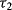# 2.4 CFA Model with Categorical Indicators

Mplus is developed on the basis of the LISCOMP,13 a SEM program that was well- suited for SEM with categorical outcomes. It is convenient to implement SEM with categorical outcome in Mplus. In this section, we extend the conventional CFA model to a CFA model with categorical indicators (binary or ordered categorical). Assuming an ordered categorical variable has M categories (m = 1, 2, . . . , M) with observed values of (U = 1), (U = 2), . . . , (U = M), and (U = 1) < (U = 2) < . . . (U = M), then there would be (M − 1) unknown thresholds that separate the adjacent categories; that is:

(2.9)where y* is an unobserved normally distributed continuous latent variable underlying the observed categorical variable U; the,, . . . , andare (M − 1) thresholds and, that link the underlying latent y* variable to the values of the observed categorical variable U.

Traditionally, the ADF estimator (Browne, 1984) is used for SEM with categorical outcomes ...

Get Structural Equation Modeling: Applications Using Mplus now with the O’Reilly learning platform.

O’Reilly members experience books, live events, courses curated by job role, and more from O’Reilly and nearly 200 top publishers.